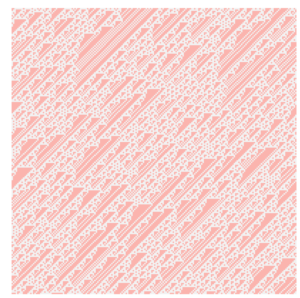# Tag Archives: Mellin Transform

## The wrong way to compute a sum: addendumIn my previous note, I looked at an amusing but inefficient way to compute the sum $$\sum_{n \geq 1} \frac{\varphi(n)}{2^n – 1}$$ using Mellin and inverse Mellin transforms. This was great fun, but the amount of work required was more intense than the more straightforward approach offered immediately by using Lambert series.

However, Adam Harper suggested that there is a nice shortcut that we can use (although coming up with this shortcut requires either a lot of familiarity with Mellin transforms or knowledge of the answer).

In the Lambert series approach, one shows quickly that $$\sum_{n \geq 1} \frac{\varphi(n)}{2^n – 1} = \sum_{n \geq 1} \frac{n}{2^n},$$ and then evaluates this last sum directly. For the Mellin transform approach, we might ask: do the two functions $$f(x) = \sum_{n \geq 1} \frac{\varphi(n)}{2^{nx} – 1}$$ and $$g(x) = \sum_{n \geq 1} \frac{n}{2^{nx}}$$ have the same Mellin transforms? From the previous note, we know that they have the same values at $1$.

We also showed very quickly that $$\mathcal{M} [f] = \frac{1}{(\log 2)^2} \Gamma(s) \zeta(s-1).$$ The more difficult parts from the previous note arose in the evaluation of the inverse Mellin transform at $x=1$.

Let us compute the Mellin transform of $g$. We find that \begin{align} \mathcal{M}[g] &= \sum_{n \geq 1} n \int_0^\infty \frac{1}{2^{nx}} x^s \frac{dx}{x} \notag \\ &= \sum_{n \geq 1} n \int_0^\infty \frac{1}{e^{nx \log 2}} x^s \frac{dx}{x} \notag \\ &= \sum_{n \geq 1} \frac{n}{(n \log 2)^s} \int_0^\infty x^s e^{-x} \frac{dx}{x} \notag \\ &= \frac{1}{(\log 2)^2} \zeta(s-1)\Gamma(s). \notag \end{align} To go from the second line to the third line, we did the change of variables $x \mapsto x/(n \log 2)$, yielding an integral which is precisely the definition of the Gamma function.

Thus we see that $$\mathcal{M}[g] = \frac{1}{(\log 2)^s} \Gamma(s) \zeta(s-1) = \mathcal{M}[f],$$ and thus $f(x) = g(x)$. (“Nice” functions with the same “nice” Mellin transforms are also the same, exactly as with Fourier transforms).

This shows that not only is $$\sum_{n \geq 1} \frac{\varphi(n)}{2^n – 1} = \sum_{n \geq 1} \frac{n}{2^n},$$ but in fact $$\sum_{n \geq 1} \frac{\varphi(n)}{2^{nx} – 1} = \sum_{n \geq 1} \frac{n}{2^{nx}}$$ for all $x > 1$.

I think that’s sort of slick.